# C++ Tutorial 4 : Exception Handling & LoopingCheat Sheet : https://goo.gl/BvEE6C
Best C++ Book : http://amzn.to/2IB3950
https://www.patreon.com/derekbanas

In this tutorial I’ll be covering While, Break, Continue, Exception Handling, Do While, Random Numbers and more complex problems for you to solve.

The problems are starting to get more complex, but don’t worry if you can’t solve them. The goal is just to get better at solving problems, which only comes from practice.

## 31 thoughts on “C++ Tutorial 4 : Exception Handling & Looping”

1.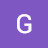Sirius 165 says:

12:18 is where exception handling is discussed.

2.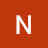Naman Mathur says:

Play it at 1.25x it's better that way

3.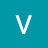Ved Bhatawadekar says:

which ide is this?

4.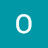OpheliaSHolmes says:

9:21 Needed that
; _;

5.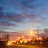Justin K says:

18:12 if only it was java.

try{
}
catch{
}
finally {
System.out.println("can you print me?n");

}

finally block always gets run, even after exceptions are thrown

6.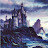Aniket Gupta says:

Is exception handling is necessary for writing in the program? At 15:32 can we directly write it as(num2 == 0){cout << "Error : Division by zero is not possible"}

7.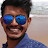Angshuman Pal Chowdhury says:

sir, What is the need of stumpspace in the program because without that my gives the exact same output ..

8.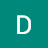Daniel Cahyo says:

I like how you use transition to divide one concept to another. It would be good if you also provide a timestamp to each concepts(But hey! The video was made in 2018. I haven't checked your newest yet)

9.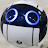TheDroidBay says:

Any clues how to get this "continuing" behaviour in Visual Studio 2015? It just jumps to a default exception and quits

10.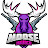MooseJuuice says:

My code to solve the Pine trees problem without hints was as follows:
#include <cstdlib>

#include <iostream>

#include <vector>

using namespace std;

vector <int> Range(int start, int end, int increment);

int main(){

int height;

int maxLength;

int gridSize;

vector<int> pinecones;

cout << "How tall is the tree : ";

cin >> height;

maxLength = (height *2) -1;

gridSize = maxLength*height;

pinecones = Range(height, maxLength, 1);

for(int k = 0; k < gridSize; k++){

if((k !=0) && (k % maxLength != 0)){

if(pinecones[k] == 0){

cout << ' ';

}

else{

cout << '#';

}

}

else if(k == 0){

cout << ' ';

}

else if(k % maxLength == 0){

cout << "n";

if(pinecones[k] == 0){

cout << ' ';

}

else{

cout << '#';

}

}

}

cout << "n";

for(int o = 0; o < maxLength; o++){

if(o != height-1){

cout << ' ';

}

else{

cout << '#';

}

}

return 0;

}

vector <int> Range(int start, int end, int increment){ //start = height // end = maxlength

vector<int> resultVec;

// vector<vector <int> vectorDUB;

for(int i = 0; i <= start; i += increment){

for(int j = 0; j < end; j+=increment){

if(j > ((start – 1)+i) || j < ((start – 1)-i)){

resultVec.push_back(0);

}

else{

resultVec.push_back(1);

}

}

}

return resultVec;

}
tips?

11.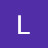Luna Dusk says:

Bit late to the party, but …. can you show how to turn your range function (and any other useful functions) into a library?

12.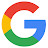Alphabet says:

<>

13.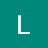Lucky Luuk says:

Hey Derek, thanks a lot for these tutorials, you rock!

Here's my solution to the pine tree problem:
void PineTrees()
{
int length = 0;

std::cout << "How tall is the tree? " << std::endl;

std::cin >> length;
int i = 0;

while (i < length)

// plan: while tree not long enough,

// print a hashtag i times

{

std::vector<int> nHashtags = Range(1, 2*i+1, 1); // determine amount of hastags in a row

std::cout << "tt"; // clear some space

for (int k = 0; k < i; k++) // For centering the tree around the middle

{

std::cout << "b";

}

for (auto j : nHashtags) // print the hastags

{

std::cout << "#";

}

std::cout << std::endl;

i++;

}

std::cout << "tt#" << std::endl; // At the end, add the stem;
}

It's about the same length as your solution, maybe slightly shorter. Hope it helps people 🙂

14.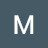Matthew Quinn says:

for the final example(number guessing game) im trying to throw an exception so that it wont crash if a user puts in a char instead of an int. Any help is greatly appreciated 🙂

15.Matthew Quinn says:

Is there a reason to type std:: instead of declaring "using namespace std;" at the beginning of the code? New to this stuff and a lot of things that should be obvious to me are not.

16.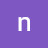nikos antoniou says:

Hi Derek this is my version

int lines;

int i; //number of rows

int y; // number of columns

int spaces;

int itteration = 0;

cout << "Inset the lines you want the tree: ";

cin >> lines;

for (i = 1; i <= lines; i++) {

for (spaces = 1; spaces <= lines – i; spaces++) {

cout << " ";

}

for (y = 0; y < i + itteration; y++) {

cout << "#";

}

itteration += 1;

cout << "n";

}

17.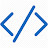Alex Weissnicht says:

This works too:

int rows = 0;
std::cout << "How many rows should the tree have";
std::cin >> rows;
for (int i = 1; i <= rows; ++i) std::cout << std::string(rows – i, ' ') << std::string(i * 2 – 1, '#') << std::endl;

18.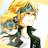Muhammad Nabeel says:

hey Derek excellent fan of your work , i was wondering what font style are you using in the IDE it looks good, could you tell me where i could download it

19.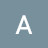Alex Johnson says:

Time to print the STOMP

20.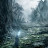Nandhan Natarajan says:

what is the difference between srand and std::rand()?

21.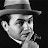pzg2008 says:

//this is my solution without range function.

int main() {

int marginalSpace = 1;

int treeHeight;

int i = 0;

int spaceTreeHeight = treeHeight;

std::cout << " how tall is the tree? n";

std::cin >> treeHeight;

while (i < treeHeight)

{

for (int k = spaceTreeHeight; k >= marginalSpace; k–)

{

printf(" ");

}

for (int j = 0; j <= i*2; j++)

{

printf("#");

}

printf("n");

i++;

spaceTreeHeight–;

}

for (int p = marginalSpace; p < treeHeight+1; p++) {

printf(" ");

}

printf("#n");

return 0;

}

22.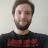Julio Cesar de Moraes Carvalho Filho says:

My Solution: I am just happy that it works haha

int main()

{

int PineSize=0,Spaces=0,c=1;

cout<<"Say size";

cin>>PineSize;

Spaces=PineSize-1;

int aux=Spaces;

while(PineSize>=0)

{

if(PineSize!=0)

{

spaces(Spaces);

PineSize–;

Spaces–;

tree(c);

}else{

spaces(aux);

cout<<"#";

break;

}

}

}

void spaces(int spaces)

{

while(spaces>0)

{

cout<<" ";

spaces–;

}

}

void tree(int &c)

{

int runs=0;

runs=c+2;

while(c>0)

{

cout<<"#";

c–;

}

cout<<endl;

c+=runs;

}

23.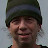Robert Schindehette says:

Hi Derek,

Terrific video as usual. You're a superhero!

Regarding the use of the Range function in this video.

I am using the GNU Compiler g++ and it compiles the code as you wrote it successfully. But I am using the -Wall option to display warnings because I think it helps me to better understand what is going on.

The compiler throws the following "unused variable" warnings:

C:/desktop\$ g++ -Wall -std=c++17 tree.cpp -o tree

tree.cpp: In function ‘int main()’:

tree.cpp:70:14: warning: unused variable ‘x’ [-Wunused-variable]

for(auto x: Range(1, spaces, 1))

^

tree.cpp:74:14: warning: unused variable ‘x’ [-Wunused-variable]

for(auto x: Range(1, hashes, 1))

^

tree.cpp:92:10: warning: unused variable ‘x’ [-Wunused-variable]

for(auto x: Range(1, stumpSpaces, 1))

^

I found a couple simple solutions to eliminate this warning and there are probably more. I just don't understand why we get the warning in the first place?

for(auto x: Range(1, spaces, 1))

{(void)x; std::cout << " ";}

for(auto x: Range(1, spaces, 1))

{x = x; std::cout << " ";}

As a side note something that seems to work like "break;" is "return 1;"

Are there any advantages or caveats to one or the other?

Thanks for all that you do!!

24.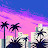Amilcar Technologies says:

Hi Derek why you have used printf in the else after the throw , we can use printf in c++ ?

25.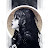Miss Fortune says:

Seeing break being used hurt my soul a little bit.

26.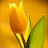Just Someone says:

Why do we have to use the throw and catch functions when exception handling to output an error warning? Can't we just use std::cout like normal?

27.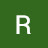Ryan Tripi says:

Another great video, I didn't realize how simple "drawing" something in programming was until looking at this video.
Question though, for the problem that you finish writing at about 11:40 , how does using the Range function work for the for loop? Is it that it counts the number of times the Range function loops until it hits either the "spaces" or "hashes" number and performs the loop that number of times?

28.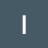Ivo van der Bruggen says:

Hey, at 15:09, why did you use const* char exp? isn't a char a single character? It can't hold that throw string right? And also why make it a pointer?

29.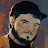Chris The Pack Rat says:

I made an alternate range function, thought it was a bit cleaner and didn't create a second vector:

void RangeVector(std::vector<int> *vec, int start, int max, int incr) {

for (int j = start; j < max + 1; j += incr) {

vec->push_back(j);
}

}

30.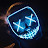Logan Elliott says:

Here is my solution to the first problem with a slightly different approach:

void DisplayTree(int height) {
int length = height * 2 – 1; // max row length
for(int i = 0; i < height; i++) {

int row = i * 2 + 1; // length of the tree row: '#####'
int offset = (length – row) / 2; // length of the spaces

for(int j = 0; j < offset; j++) std::cout << " ";
for(int j = 0; j < row; j++) std::cout << "#";

std::cout << std::endl;
}

int offset = (length – 1) / 2; // length of the spaces for the stump

for(int i = 0; i < offset; i++) std::cout << " ";
std::cout << "#" << std::endl;

}

31.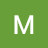Mohanakrishna Vanamala Hariprasad says:

Hi Derek,

Will this be considered cheating ? I didn't make use of the range function we created.

#include <iostream>
#include <string>

using namespace std;

/*
How tall is the tree : 5
#
###
#####
#######
#########
#

Idea:
1. Decrement spaces by one each time through the loop
2. Incerement the hashes by 2 each time
3. Save spaces to the stump by calculating tree height – 1
4. Decrement the tree height until equals zero
5. Print spaces and the hashes for each row
6. Print stump spaces and then 1 hash

*/

int main() {
int treeHeight, spaces, hashes, stump;
cout << "Enter the height of the tree : ";
cin >> treeHeight;
stump = treeHeight – 1;
spaces = treeHeight – 1;
hashes = 1;
while (treeHeight > 0) {
cout << string(spaces, ' ') << string(hashes, '#') << "n";

treeHeight -= 1;
hashes += 2;
spaces = treeHeight – 1;
}

cout << string(stump, ' ') << string(1, '#');
cout << "n";
return 0;
}